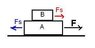# Work done by static friction

• krugmik
In summary, static friction can do positive, negative, or zero work, as well as nonnegative work. This is because in certain situations, such as when the whole system moves to the left or when a rolling ball has zero displacement, the work done by static friction can be considered to be zero. It is important to consider the specific circumstances and wording of the question in order to determine the correct answer.

## Homework Statement

"The work done by static friction can be:
a) positive
b) negative
c) zero
d) nonnegative
e) any of the above

## Homework Equations

W=F*d
F(friction)= μ *FN

## The Attempt at a Solution

I thought the answer must logically be "c) zero" because static friction has no distance for as soon as movement begins static friction ends and as such there can be no work without distance. This is for a test correction and so my teacher has told me the correct answer is "e) any of the above" but I must explain why. I think it must have something to do with the wording of the question or a special circumstance in which static friction is considered to do work.

See attachment. There is enough static friction between the blocks so they accelerate together. The only force acting on block B is Fs. What is the work of Fs on B if the whole system moves to the right by a distance D?
At the same time, the same static friction force with opposite sign acts on block A. What work does the static friction do on it?
It can happen that the whole system moves to the left, but the external force accelerates the system to the right. What is the work of the friction on the bodies?

For a rolling ball, the displacement is really zero, so the work of Fs is zero.

ehild

#### Attachments

•statfri.JPG
4 KB · Views: 1,198